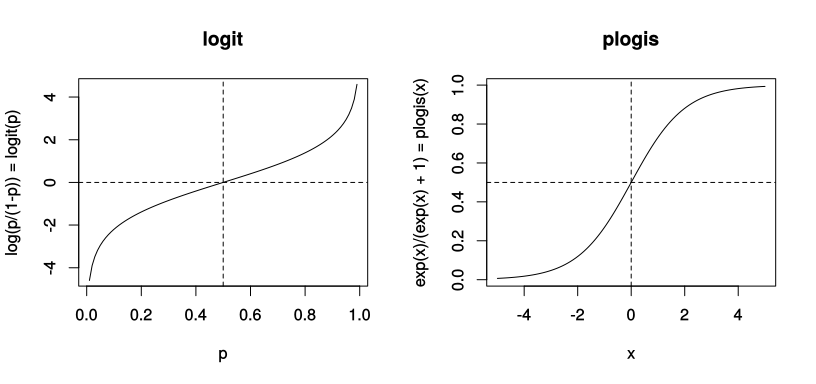Martijn Wieling
University of Groningen

This lecture

• Introduction
• Logistic regression
• Standard Italian and Tuscan dialects
• Material: Standard Italian and Tuscan dialects
• Methods: R code
• Results
• Discussion

Logistic regression

• Dependent variable is binary (1: success, 0: failure), not continuous
• Transform to continuous variable via log odds: $$\log(\frac{p}{1-p})$$ = logit$$(p)$$
• Automatically in GAM by setting family="binomial"
• Transformation of dependent variable: generalized additive model
• interpret coefficients w.r.t. success as logits: in R: plogis(x)Standard Italian and Tuscan dialects

• Standard Italian originated in the 14th century as a written language
• It originated from the prestigious Florentine variety
• The spoken standard Italian language was adopted in the 20th century
• People used to speak in their local dialect
• We investigate the relationship between standard Italian and Tuscan dialects
• We focus on lexical variation
• We use social, geographical and lexical variables

Material: lexical data

• We use lexical data from the Atlante Lessicale Toscano (ALT)
• We focus on 2060 speakers from 213 locations and 170 concepts
• Total number of cases: 384,454
• Binary dependent variable:
• 1: lexical form was different from standard Italian
• 0: lexical form was identical to standard Italian

Geographic distribution of locationsMaterial: predictors

• Speaker age
• Speaker gender
• Speaker education level
• Speaker employment history
• Number of inhabitants in each location
• Average income in each location
• Average age in each location
• Frequency of each concept

library(mgcv)